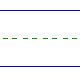Name: ______________________________________________ Date: _____________

Multiplication

Instructions: Multiply.

 6 x 2 =3 x 2 =0 x 2 =2 x 8 =2 x 3 =4 x 2 =2 x 9 =2 x 7 =2 x 1 =8 x 2 =9 x 2 =2 x 0 =7 x 2 =2 x 4 =1 x 2 =2 x 5 =2 x 2 =2 x 6 =